# Change Line Type of Base R Plot (6 Examples)

This article illustrates how to modify the lines types in a plot in the R programming language.

The article contains the following contents:

It’s time to dive into the R syntax…

## Introducing Example Data

Consider the following example data:

```x <- 1:10                                  # Create example data
y <- c(3, 1, 7, 2, 3, 5, 5, 1, 8, 4)```

The previous R code illustrates that our example data are two numeric vectors containing ten elements each.

As a next step, we can draw a plot of our data:

`plot(x, y, type = "l")                     # Draw default line plot`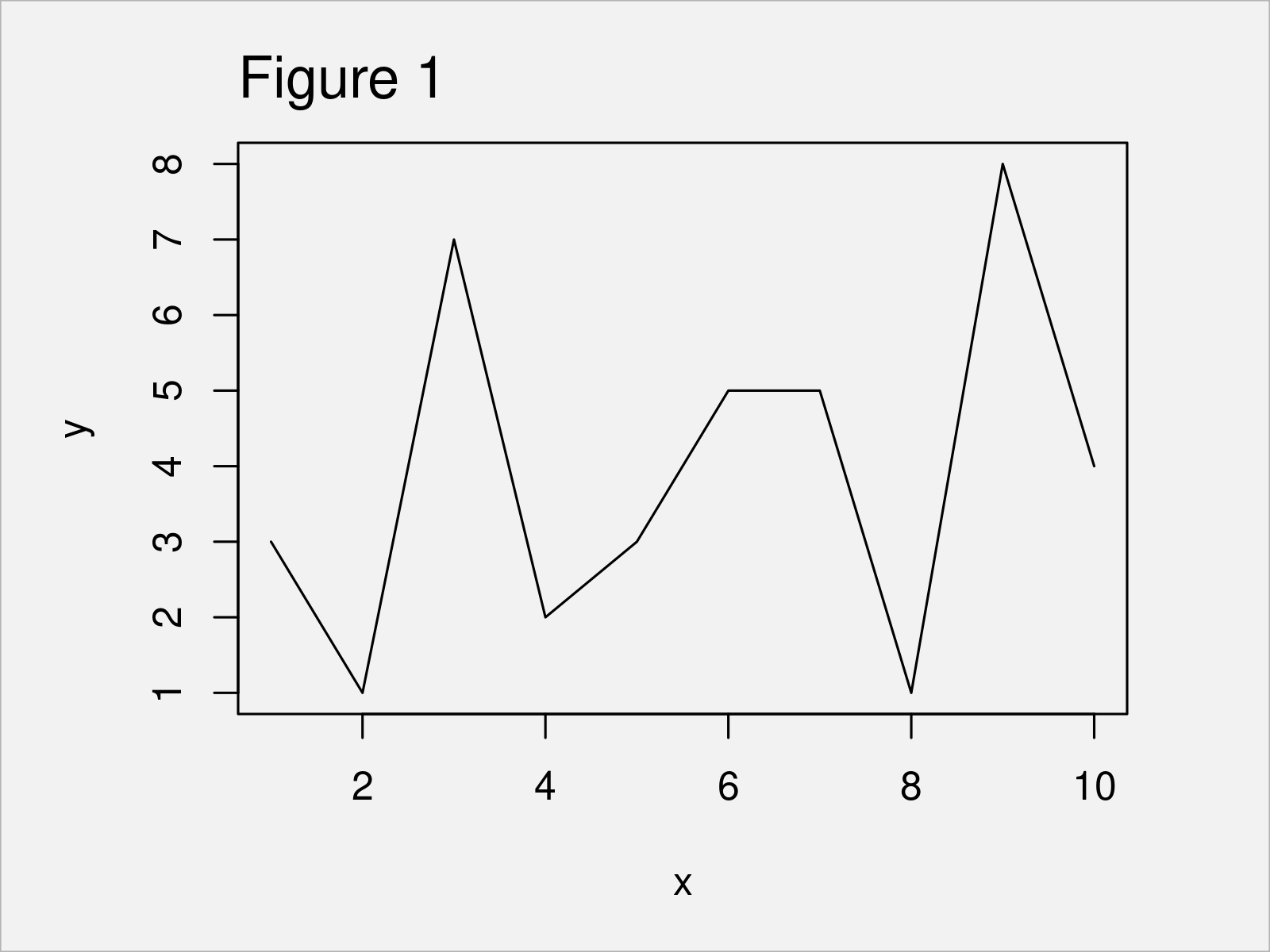As shown in Figure 1, the previous code has created a line plot with default line type (i.e. a solid line with the color black).

In the following examples, I’ll explain how to change the shape of this line using the lty argument of the plot function.

## Example 1: Draw Plot with Dashed Line

```plot(x, y, type = "l",                     # Dashed line
lty = "dashed")```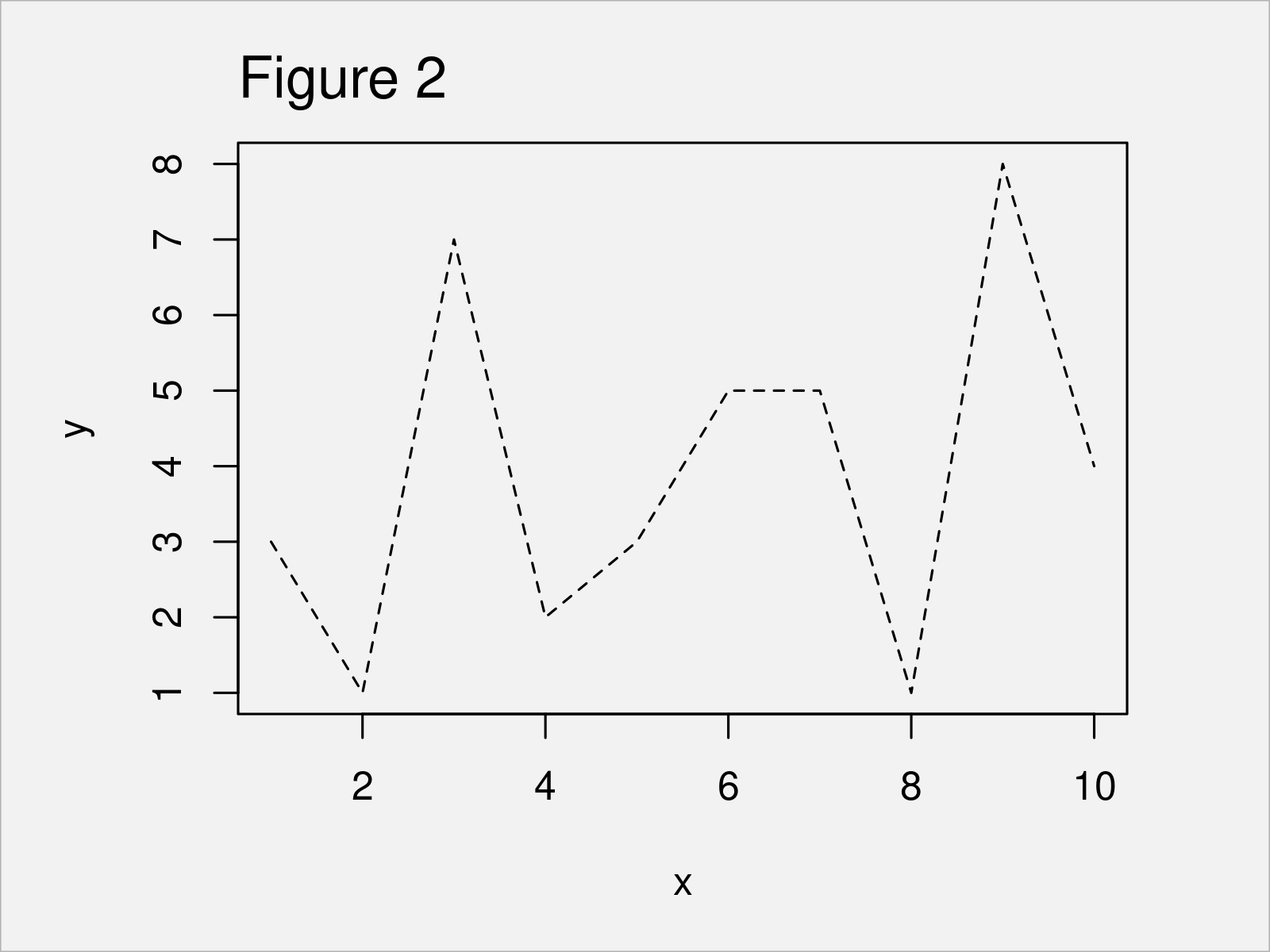## Example 2: Draw Plot with Dotted Line

```plot(x, y, type = "l",                     # Dotted line
lty = "dotted")```## Example 3: Draw Plot with Dotdash Line

```plot(x, y, type = "l",                     # Dotdash line
lty = "dotdash")```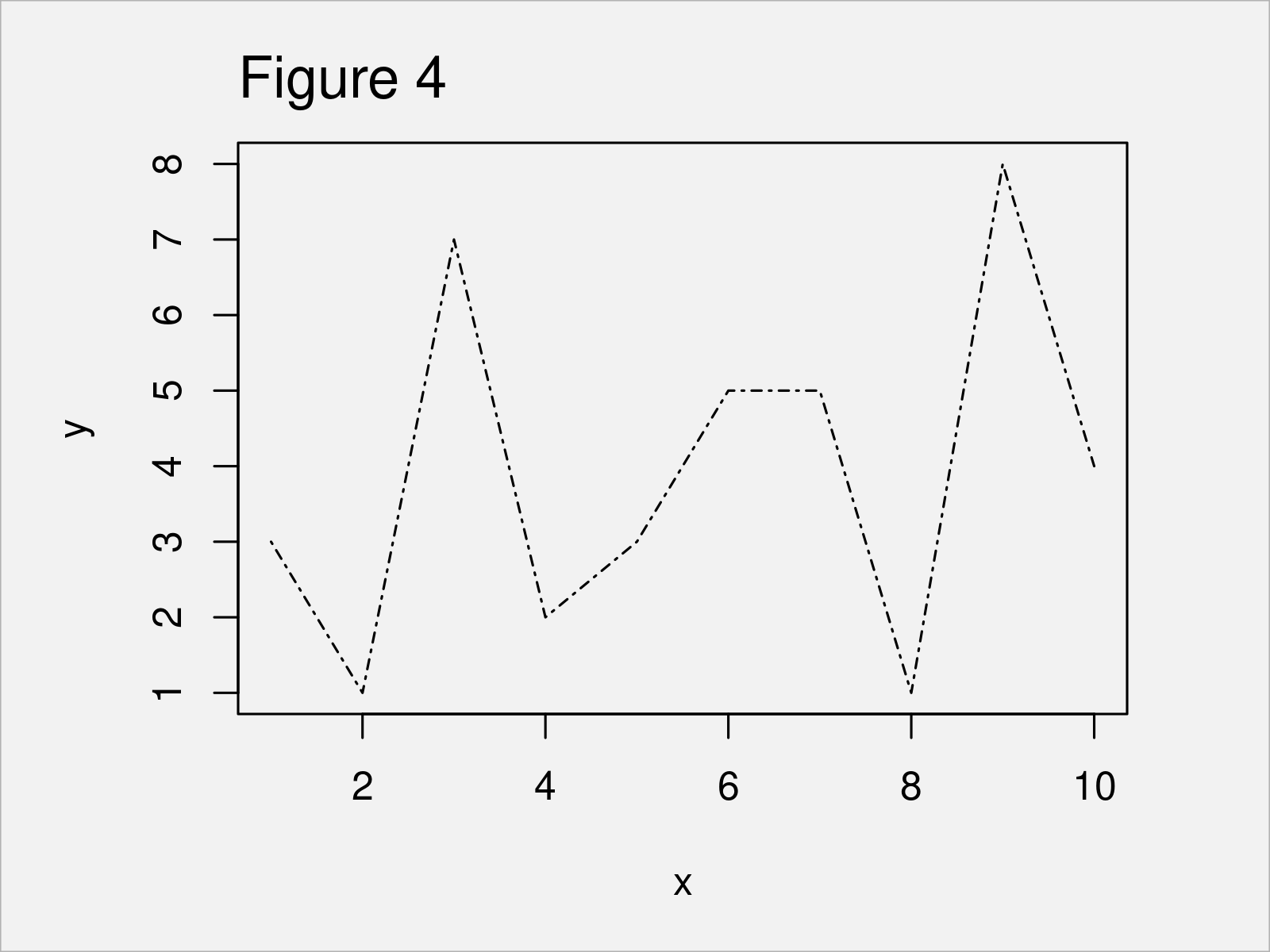## Example 4: Draw Plot with Longdash Line

```plot(x, y, type = "l",                     # Longdash line
lty = "longdash")```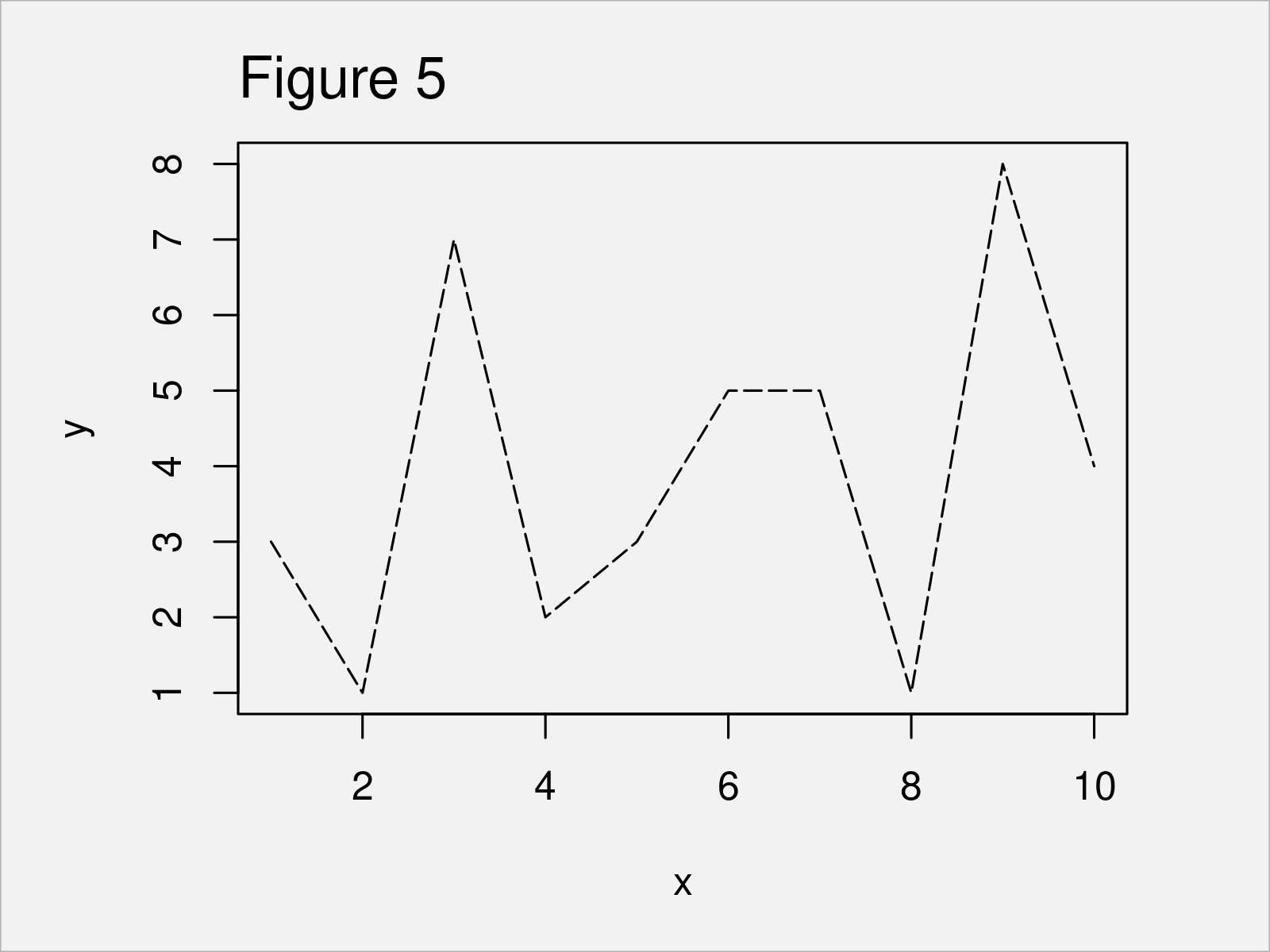## Example 5: Draw Plot with Twodash Line

```plot(x, y, type = "l",                     # Twodash line
lty = "twodash")```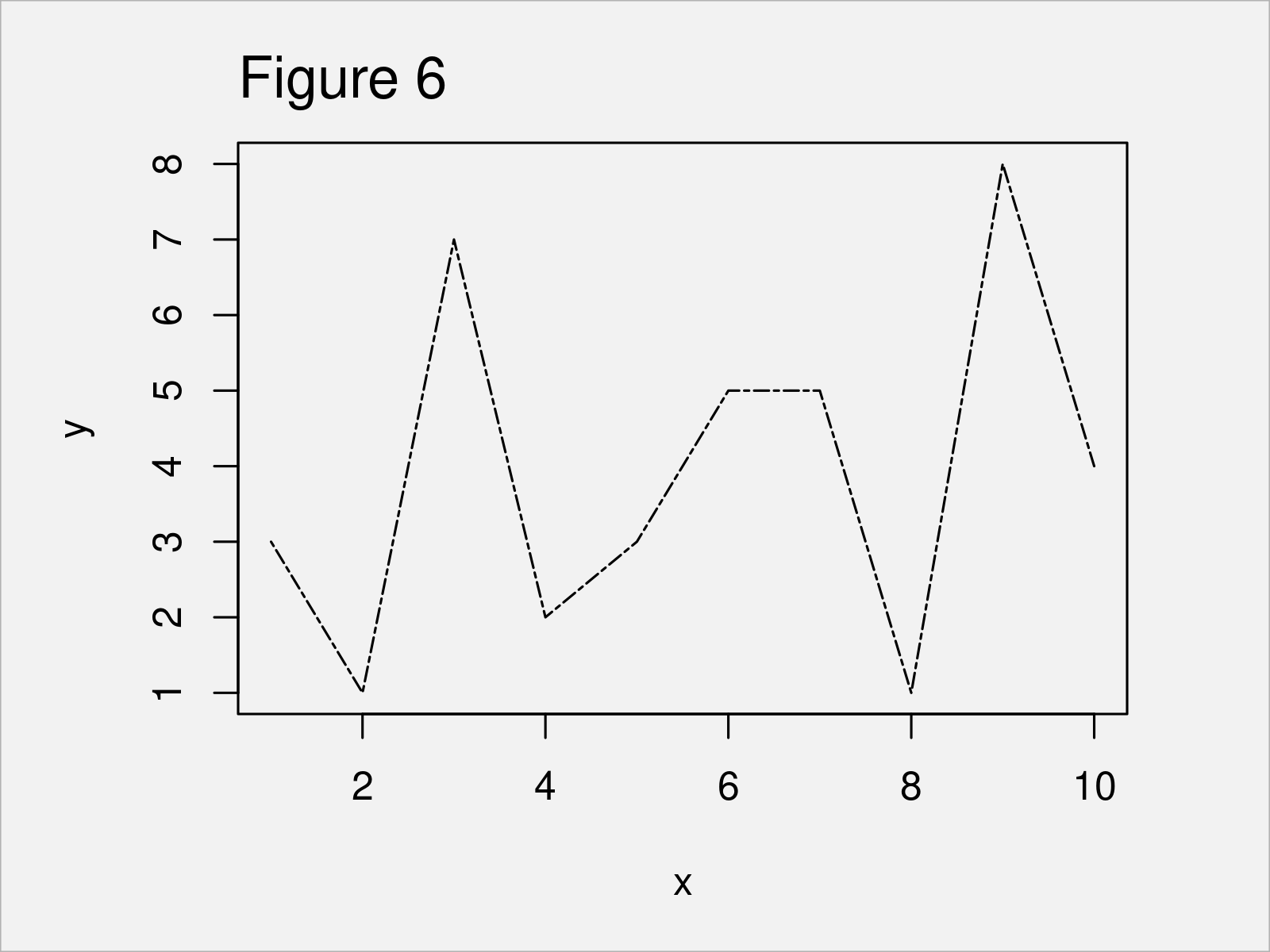## Example 6: Draw Plot with Multiple Line Types

The following syntax demonstrates how to draw all previously shown line types in a single plot.

For this task, we can use the plot and lines functions as shown below. The following syntax is also adding a legend to our graphic, which identifies the different commands for each of the lines.

```plot(x, y, type = "l", ylim = c(1, 15))    # Draw all line types in one plot
lines(x, y + 1, type = "l", lty = 2, col = 2)
lines(x, y + 2, type = "l", lty = 3, col = 3)
lines(x, y + 3, type = "l", lty = 4, col = 4)
lines(x, y + 4, type = "l", lty = 5, col = 5)
lines(x, y + 5, type = "l", lty = 6, col = 6)
legend("topleft",
c("solid", "dashed", "dotted", "dotdash", "longdash", "twodash"),
col = 1:6,
lty = 1:6)```## Video, Further Resources & Summary

Please accept YouTube cookies to play this video. By accepting you will be accessing content from YouTube, a service provided by an external third party.If you accept this notice, your choice will be saved and the page will refresh.

Furthermore, you could have a look at the related articles on this homepage.

In this R tutorial you have learned how to change the lines types in a graphic. Let me know in the comments section, in case you have any further questions.

Subscribe to the Statistics Globe Newsletter